## Part Two of a Three-Part Series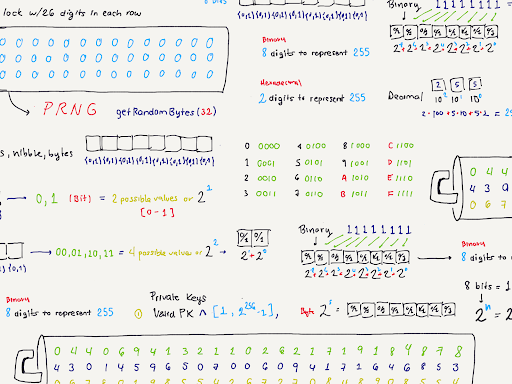Disclaimer: Please note that all the private keys generated and used in this blog are for educational purposes only. Do not use any of the code, keys, or addresses shared in this post to hold any kind or amount of crypto assets.

## Private keys are building blocks

As we reviewed in Part 1 of our mini-series, “Unlocking the Mysteries of  Private Keys,” the procedure for generating private keys relies on pseudo-random number generators (PRNG) with enough entropy. The most important thing to remember about a private key is that it needs to be selected randomly from the integer space 2^256-1. Any number can be a private key as long as it’s within the value of 1 and 2^256 - 1.

Now that we understand a bit of the mathematics behind private keys, we can go ahead and generate our own valid private key. A good way to visualize this generation process is to think of a horizontal digit combination lock that is 78 digits long (the total amount of digits in 2^256-1) and then to split it into three rows of 26 digits each. You can think of a PRNG function as something that would “shuffle” all of the digits on that combination lock randomly: starting them all at 0, and subsequently generating a number without any distinguishable pattern. Let’s assume we run a PRNG function on our lock and obtain the following numbers in each row:

(1)04406941321102621719184878,(2)43014596507006094171646853, (3)06780198554267270848908554.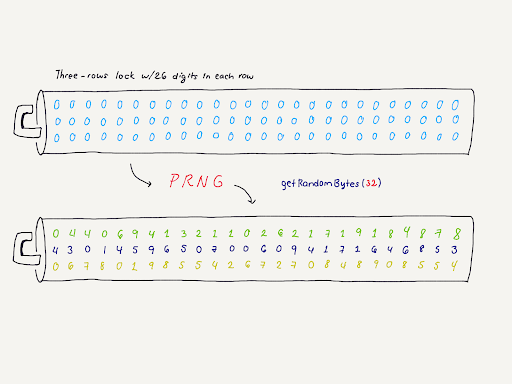Browsers use the Web Cryptography API, a modern API that provides us with cryptographic primitives such as Crypto.getRandomValues(32)(or the equivalent of creating a Buffer of 32-bytes) as a PRNG, which is seeded with your computer entropy source to generate random numbers. You should always use a digital source for random number generation, as humans are terrible at picking random numbers, as a few studies indicate.

You have just generated the private key 44069413211026217191848784301459650700609417164685306780198554267270848908554. We can now use it to generate a Bitcoin or Ethereum address or an address for nearly any blockchain that supports private keys in the 2^256-1 range.

To generate an Ethereum address from this private key, we need to do Elliptic Curve point multiplication. This can be complicated, so to simplify, we will use a computer to do it for us. First, we must understand that computers do not process information in decimal format. Computers only understand binary code, and so far, we only have our private key in a decimal numeric form. Thus, to use our private key, we must first convert it into bits and bytes.

## A short bit on bits and bytes

Just what are “bits and bytes”? Digital devices can only understand information using the numbers 0 and 1, commonly known as bits (or, “binary digit,”). Although our smartphones and computers can display characters, images, songs, etc., computers ultimately represent and process everything as bits. Groups of bits represent larger things, but from a computer’s frame of reference, they are always just a bunch of 0s and 1s. The most common representation of bits are bytes, which are composed of 8 bits. Another popular but less-used representation are nibbles, which are composed of 4 bits.

Depending on the context, multiple bits can represent characters (e.g., the letter a can be defined as 01100001 using ASCII encoding by using 1 byte) or numbers (i.e., 01100001 represents the number 97 in decimal format). In binary format, both bits and bytes can represent integers by adding the powers of all bits used, where the base is 2, and each sequential digit increases the exponent used. For instance, we usually count in decimal format, where numbers are expressed as the sums of the powers of all digits used, using the number 10 as a base. However, using a binary form, we can express numbers as 2 to the nth power, where “n” is the number of bits needed to represent and store this information in a computer.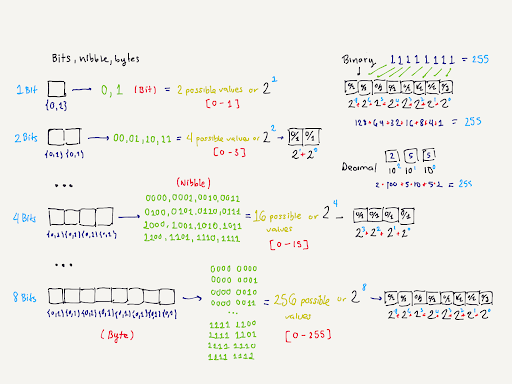8-bit video games could only express values up to 255 because the computer processing units (CPUs) used in them could only perform operations up to 8 bits.

Although we can represent any number in binary format, this format is quite “clunky.” To just express 97, we needed eight binary digits. Binary numbers are easy for computers to process but incredibly cumbersome for humans to read. So, instead of representing data in binary format, computers usually use the hexadecimal format: a positional numeral system representing numbers using a base of 16. Unlike the binary format, we can represent 4 bits in a single letter in hexadecimal format. We can represent 01100001—the number 97—by using 61, reducing six digits from our previous example. Hexadecimal numbers use ABCDEF to represent 10 to 15 and are commonly used for expressing data in small chunks.

## Counting up bits in a key

Coming back to our private key, we know it’s a number within 1 and 2^256-1. How can we represent it in bits, and how many bits do we need? We discussed that in binary format, numbers represent integers by adding the powers of all bits used, where the base is 2; thus, using 8 bits, we can represent 2^7 + 2^6 + 2^5 + 2^4 + 2^3 + 2^2 + 2^1 + 2^0, which is 255. We can then see that in 2^n, n equals the total amount of bits needed to represent any number in bits. If we correlate this reasoning, we can say that 256 bits, or even better, 32 bytes (256/8), are needed to represent our private key.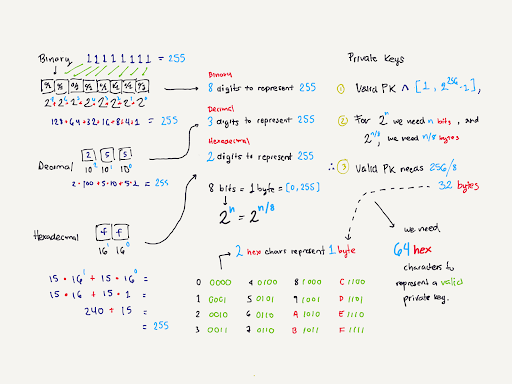Hexadecimal representation of data is meant to reduce the number of digits needed to represent numbers. Computers, however, still just process data using 0s and 1s.

If we can agree that we need 32 bytes to represent our [1, 2^256-1] private key, then using the hexadecimal format, we can agree that we need 64 characters to represent our private key. We can now convert our original private key 44069413211026217191848784301459650700609417164685306780198554267270848908554 to its equivalent hexadecimal format: 616E6769652E6A6A706572657A616775696E6167612E6574682E6C696E6B0D0A. See the additions of the letters A, B, C, D, E, and F in our new number; the presence of these letters is an easy way to identify that a number is represented in hexadecimal format.

## From private key to public key

We can now tell our computer about our private key by using its hexadecimal format. Using programming languages like JavaScript, we can easily import our private key into a format we can use for further multiplication. In the following code, we defined our private key (“sk” for secret_key, a standard notation used in cryptography) to import the hexadecimal value previously defined. We provide the hexadecimal format by ensuring the radix (base) is 16.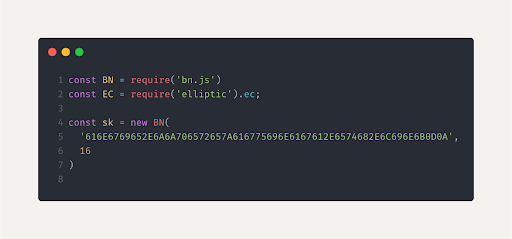Using the BigNumber library, we can ensure no decimals get lost in the conversion. These numbers are usually expressed as exponentials (e.g., 4.406941321102622e+76) and, when parsed to hexadecimal directly, lose precision. Without BigNumber, our hexadecimal conversion would return 616e6769652e6c00000000000000000000000000000000000000000000000000 instead of our actual hexadecimal number.

With our key imported, the next step is to create the public key. As you might recall from our first blog, we need to derive the public key from our private key before we can get the Ethereum address. Following the instructions from the original yellow paper from Ethereum, we found that the key generation process follows a standard ECDSA public key generation, where we multiply the generator point and concatenate the coordinates into a single value. Our public key (now defined as pk) can now be used to generate our Ethereum address.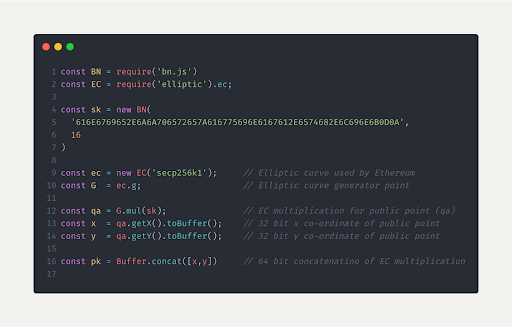The values x and y are obtained from the elliptic curve point multiplication by our private key (sk). Although a private key can be used in any blockchain as a unique identifier of an address, Ethereum is specific about using Elliptic curve secp256k1 for public key generation, and thus, their equivalent signing operations.

Alas, the final step has come. With our public key defined, we can then execute the last instruction from the yellow paper, defined as follows:

For a given private key, the Ethereum address A is defined as the rightmost 160-bits of the Keccak hash of the corresponding ECDSA public key.

Considering we already have our ECDSA public key, the only remaining task is to run the Keccak hashing function on our public key and obtain the rightmost 160-bits from this operation. As we store these operations in “buffers” (think of small boxes where we store bytes of information), we can simply “drop” (slice) the first 24 characters, leaving only 40 characters, or more concretely, 20 bytes—the size of an Ethereum address.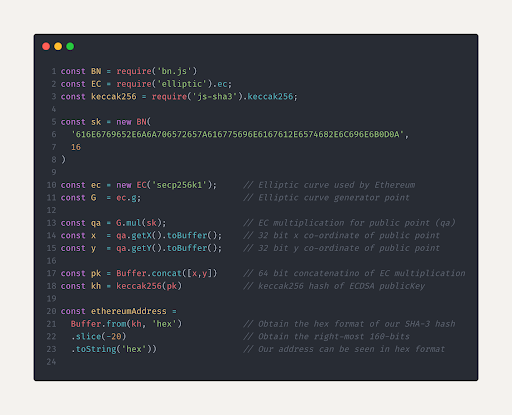Ethereum addresses are 20 bytes long by design. By dropping some of its bytes (12 to be precise), one could argue that there might be a collision where two private keys end up generating the same Ethereum address. However, as of today, that has yet to happen.

## You have created your own wallet

As you can see, from a single number (albeit a long one), you can obtain an Ethereum address where you can hold all sorts of assets: from NFTs representing music, tickets, etc., to crypto-assets that can accrue monetary value. Your Ethereum address is public, similar to your physical address, and it connects you to that unique private key.

In case you don’t want to go through the steps outlined here, you can sign up for an account on www.ShapeShift.com to create your own private key, known only to you, and leverage a number of software and hardware wallet options.

In the next and final part of our mini-series, we’ll see how we can now use our private keys to create and broadcast transactions from our Ethereum address and sign messages, and learn the implications these signatures can have in the Ethereum ecosystem.